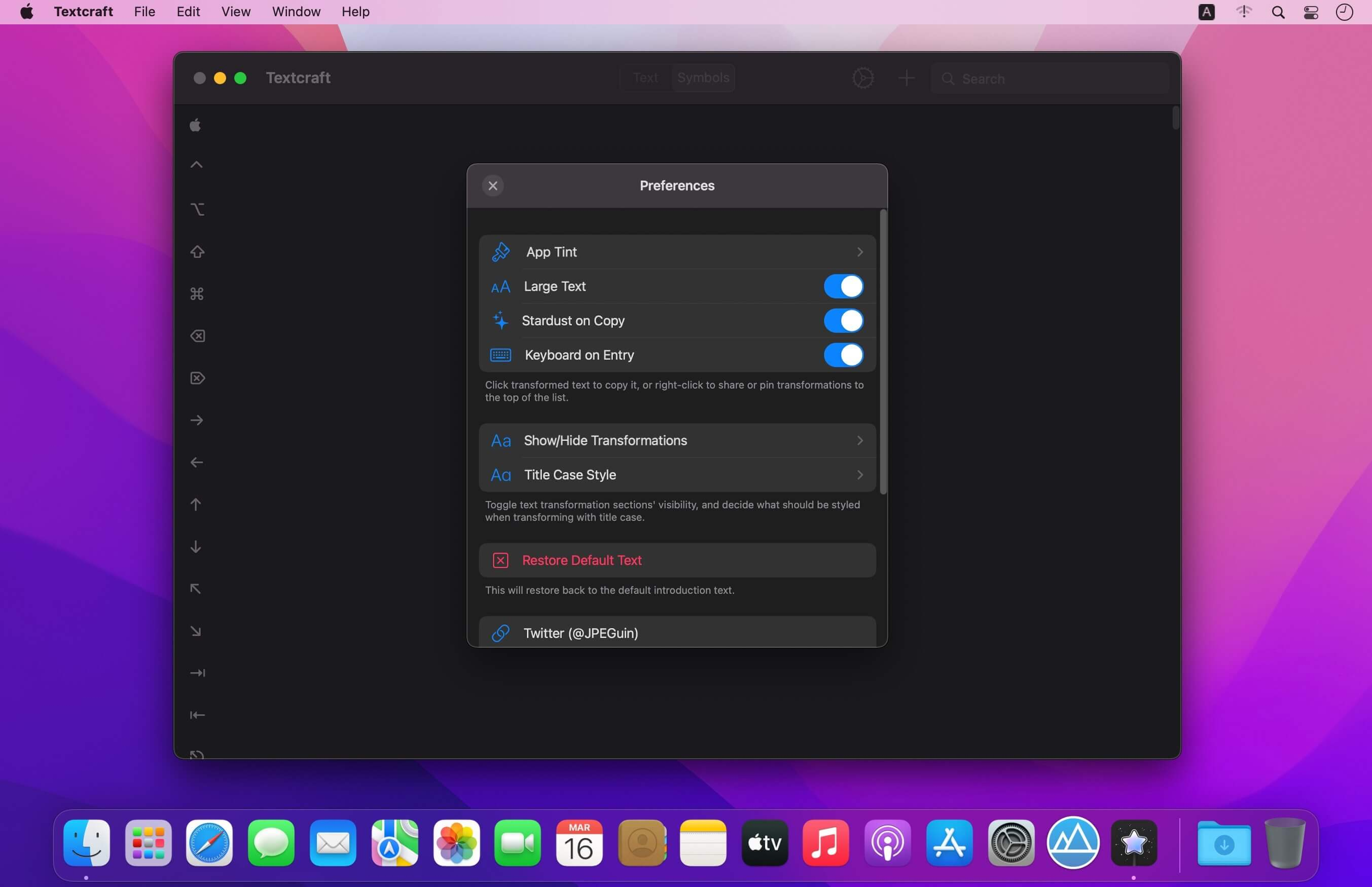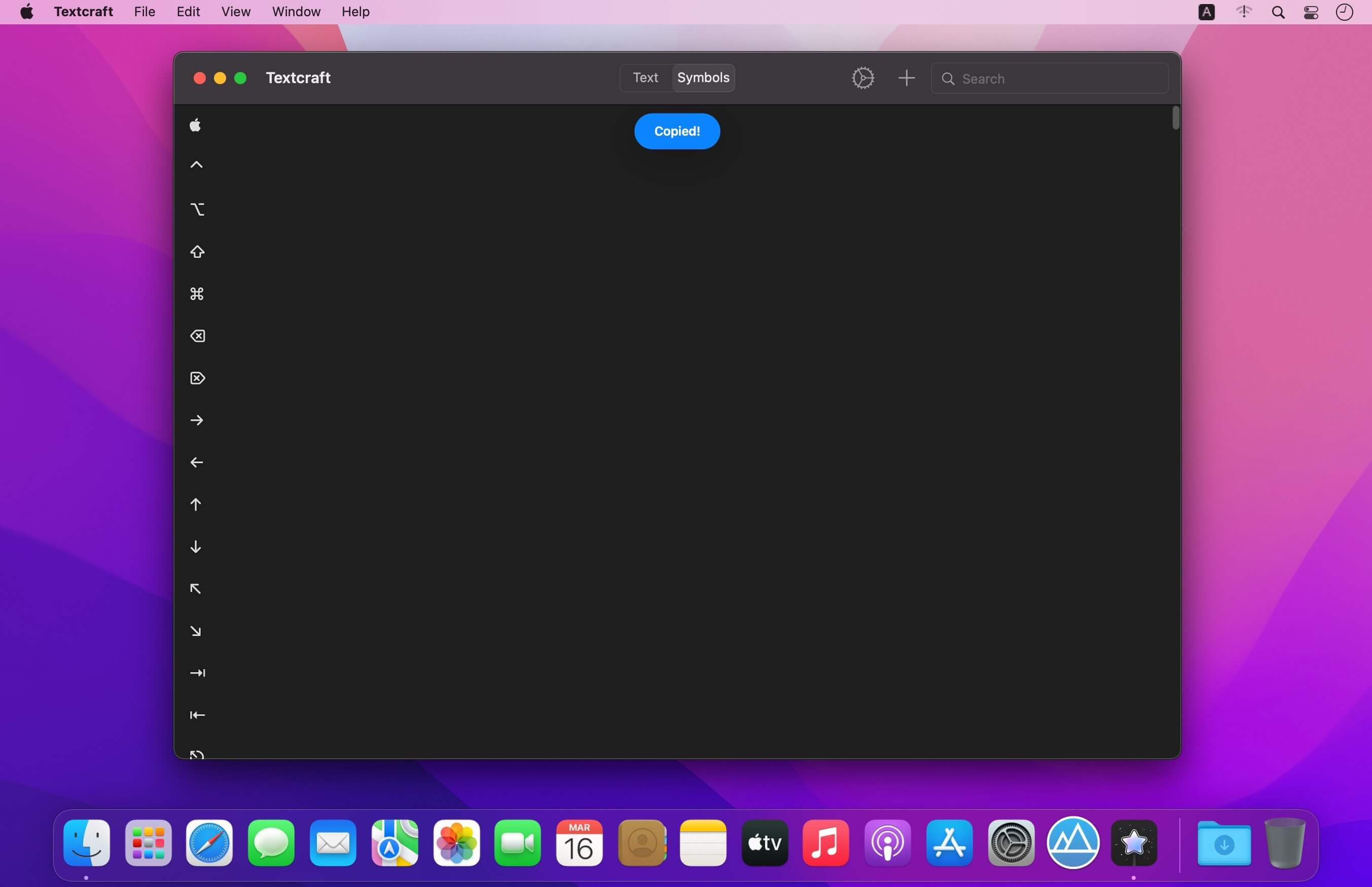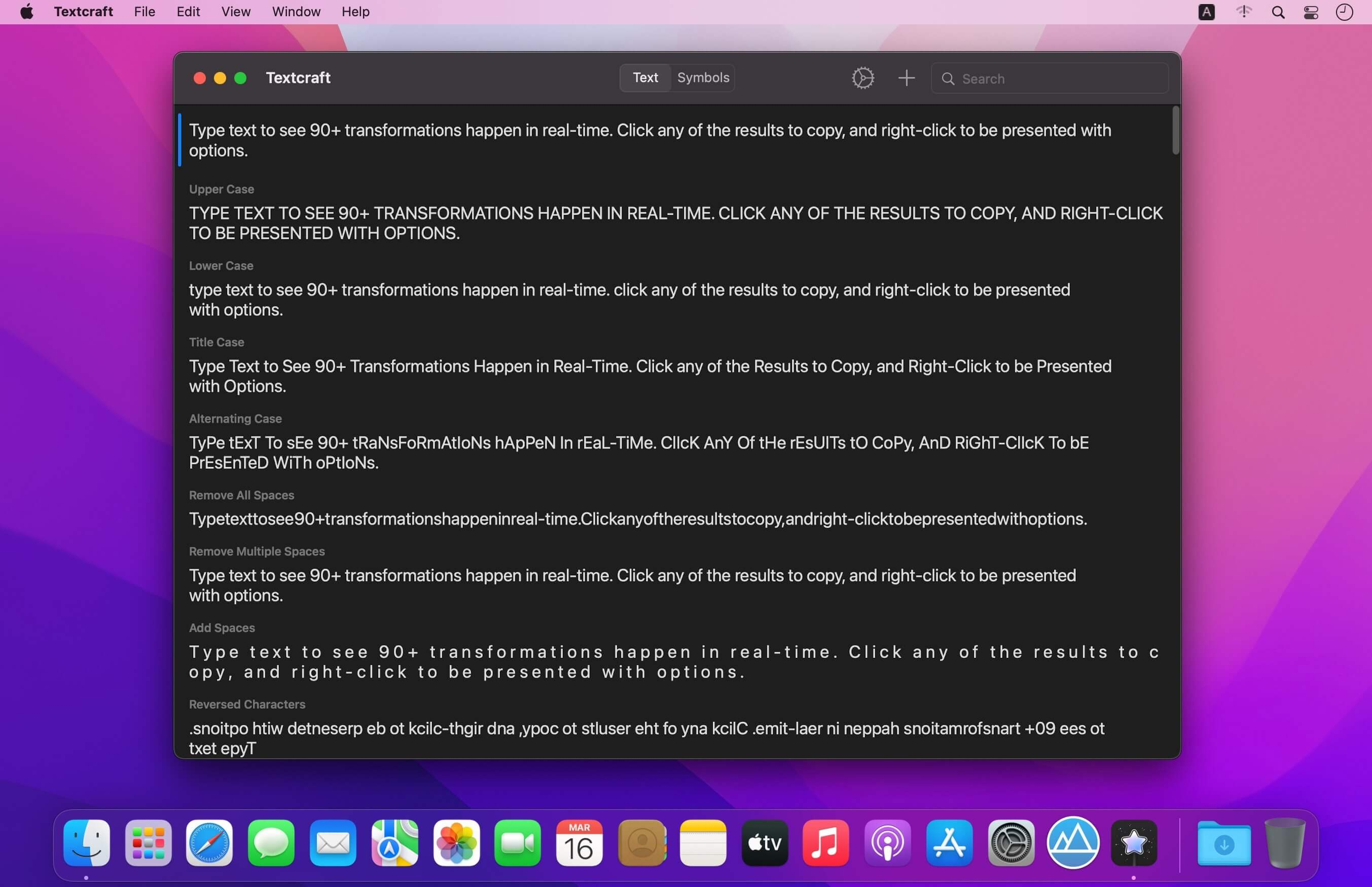Textcraft 是一个神奇的实用程序，可让您将任何文本实时转换为 90 多种不同的格式。

• 大写
• 小写
• 单词大小写
• 交替案例
• 删除所有空格
• 删除多个空格
• 添加空格
• 反转字符
• 颠倒的词
• 用下划线替换空格
• 用空格替换下划线
• 用破折号替换空格
• 用空格替换破折号
• 用破折号替换下划线
• 用下划线替换破折号
• 缩写
• 带点分隔的缩写
• 带有破折号分隔的首字母
• 带下划线分隔的首字母
• ROT13
• 大胆的
• 斜体
• 强调
• 删除线
• 斜线穿越
• 上下翻转
• 气泡
• 填充气泡
• 正方形
• 填充正方形
• 草书
• 哥特
• 多用途车
• 恶魔
• 撒旦的
• 用 Claps 替换空格
• 用表情符号替换单词
• 删除表情符号
• 删除标点符号
• 分类
• 标签
• 句号
• 删除链接
• 剥离 HTML
• 网址编码
• 网址解码
• Base64 编码
• Base64 解码
• 用加号替换空格
• 用空格替换加号
• SHA256 十六进制
• SHA512 十六进制
• 删除逗号
• 删除单引号
• 删除双引号
• 使用逗号展平列表
• 使用句号展平列表
• 使用管道展平列表
• 使用下划线展平列表
• 使用加号展平列表
• 使用正斜杠展平列表
• 使用反斜杠展平列表
• 用双引号替换单引号
• 用单引号替换双引号
• 删除元音
• 删除辅音
• 删除号码
• 虚线到项目符号列表
• 项目符号到虚线列表
• MD5 哈希
• 添加逗号
• 用 \\n 替换换行符
• 单词周围的单引号
• 单词周围的双引号
• 转换为虚线列表
• 转换为项目符号列表
• 将逗号转换为虚线列表
• 将逗号转换为项目符号列表
• 十六进制转二进制
• 二进制转十六进制
• 1337
• 上标
• 删除换行符
• 删除换行符和段落符
• 随机案例
• 句子案例
• 修剪空白
• 修剪空白和新行
• 小帽子
• 闪电
• 双闪电